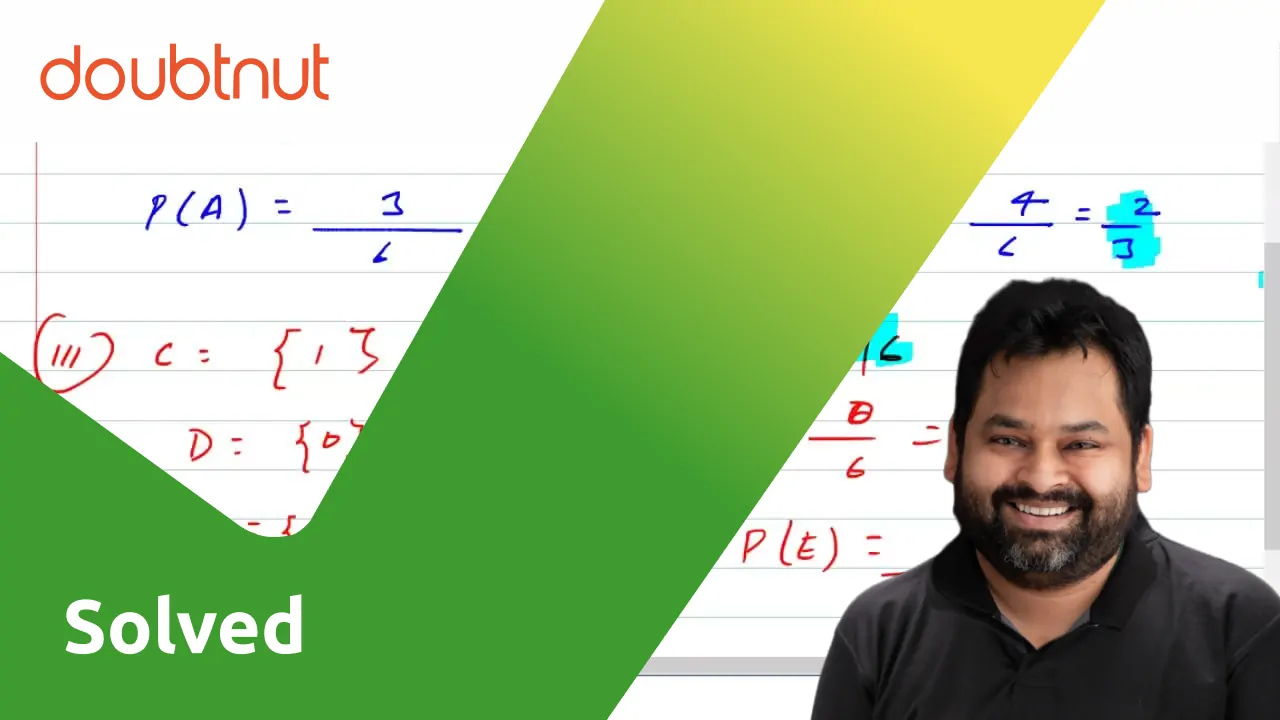Doubtnut App - Get 50 Lakh+ video solutions better than GoogleInstall Now

# A die is thrown, find the probability of following events: (i) A prime number will appear, (ii) A number greater than or equal to 3 will appear, (iii) A number less than or equal to one will appear, (iv) A number more than 6 will appear, (v) A number less than 6 will appear.

Text Solution
Verified by Experts

## In a throw of a die Samle space S={1,2,3,4,5,6} ⇒n(S)=6 (i) Let A= event of getting a prime number ={2,3,5} ⇒n(A)=3 ∴ Probability of event A is P(A)=n(A)n(S)=36=12 (ii) Let B= event of getting a number 3 or greater ={3,4,5,6} ⇒n(B)=4 ∴ Probability of event B is P(B)=n(B)n(S)=46=23 (iii) Let C= event of getting a number 1 or smaller ={1} ⇒n(C)=1 ∴ Probability of event C is P(C)=n(C)n(S)=16 (iv) Let D= event of getting a number greater than 6 ={} ⇒n(D)=0 ∴ Probability of event D is P(D)=n(D)n(S)=06=0 (v) Let E= event of getting number smaller than 6 ={1,2,3,4,5} ⇒n(E)=5 ∴ Probability of event E is P(E)=n(E)n(S)=56